Checkout JEE MAINS 2022 Question Paper Analysis : Checkout JEE MAINS 2022 Question Paper Analysis :

# Volume of a Prism

A prism is a polyhedron in which all the faces are flat, and the bases are parallel to each other. It is a solid object with flat faces, identical ends, and the same cross-section along with its length. In Geometry, we will learn the different types of prisms, such as triangular prism, pentagonal prism, and hexagonal prism. Since it is a three-dimensional shape, a prism has a surface area and volume. In this article, we are going to discuss the volume of a prism, its formulas and solved problems.

## What is the Volume of a Prism?

The volume of a prism is defined as the total space occupied by the three-dimensional object. Mathematically, it is defined as the product of the area of the base and the length.

Therefore,

The volume of a Prism = Base Area × Length

The measurement unit used to represent the volume of a three-dimensional object is cubic units.

## Volume of a Prism Formula

Now, let us discuss the volume of the different prism formulas, such as the volume of the triangular prism, rectangular prism, pentagonal prism, and so on.

### Volume of a Triangular Prism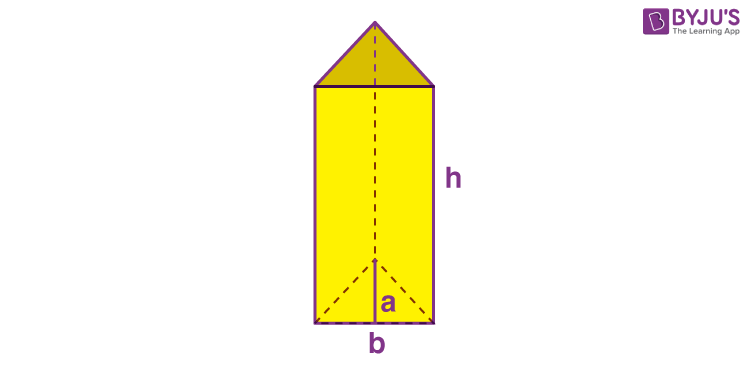A triangular prism is a prism that has three rectangular faces and two triangular bases. Since the cross-section of the triangular prism is a triangle, the formula for the volume of a triangular prism is given as:

The volume of a Triangular Prism = (½) abh cubic units.

Where

a = Apothem length of a triangular prism

b = Base length of a triangular prism

h = height of a triangular prism

### Volume of a Rectangular prism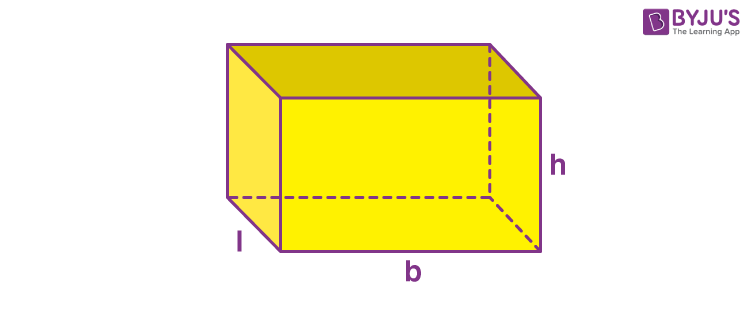A rectangular prism has four rectangular faces and two parallel rectangular bases. We know that the cross-section of a rectangular prism is a rectangle. The rectangular prism is also known as a “Cuboid”.

Hence, the formula to find the volume of a rectangular prism is given:

The volume of a Rectangular Prism = l.b.h cubic units.

Where

l = Base width of a rectangular prism

b = Base length of a rectangular prism

h = height of a rectangular prism

### Volume of a Pentagonal Prism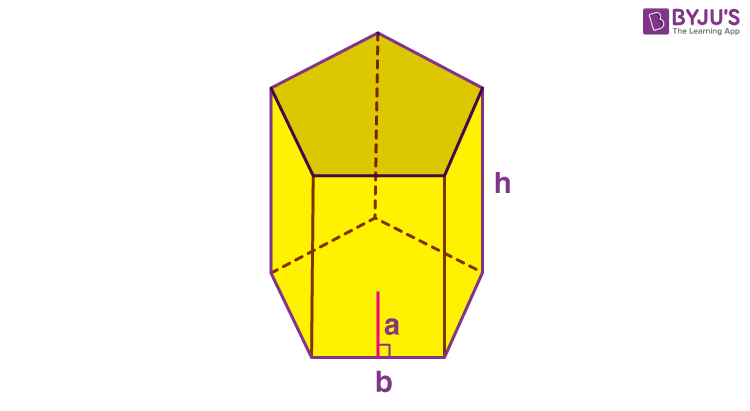A pentagonal prism has five rectangular faces and two parallel pentagonal bases. Since the base area of the pentagonal prism is (5/2) ab, the volume of the pentagonal prism is given as:

The Volume of a Pentagonal Prism = (5/2) a.b.h cubic units

Where,

a – Apothem length of the pentagonal prism.

b – Base length of the pentagonal prism.

h – Height of the pentagonal prism

### Volume of a Hexagonal Prism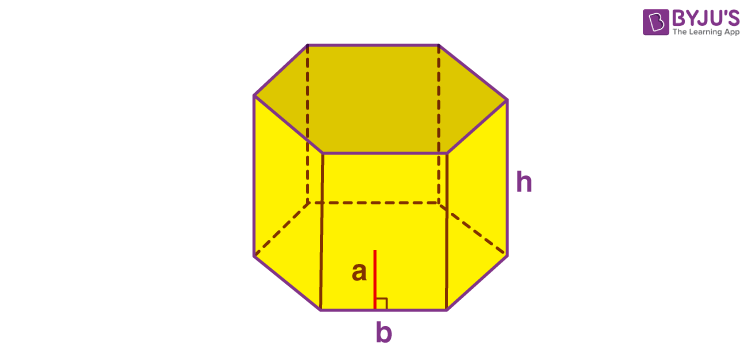A hexagonal prism is a prism with six rectangular faces and two parallel hexagonal bases. The base area of the hexagonal prism is 3ab, the formula to find the volume of a hexagonal prism is given as:

The volume of a Hexagonal Prism = 3abh cubic units

Where

a – Apothem length of the hexagonal prism.

b – Base length of the hexagonal prism.

h – Height of the hexagonal prism.

### Volume of a Prism Example

Question 1:

What is the volume of a triangular prism with dimensions of 12 m, 16 m and 20 m as given in figure.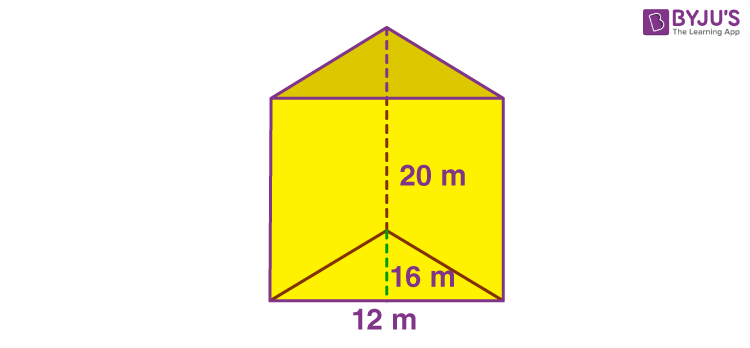Solution:

The volume of a triangular prism can be found by V = Area of base × Height of Prism

As the base is triangular, so,

Area of triangle = ½ × base × height = ½ × base × height =½ × 12 × 16 = 96

So, Volume of prism = 96 × 20 = 1920 cubic meter.

## Frequently Asked Questions – FAQs

### What is the formula for the volume of the prism?

The volume of a Prism = Base Area × Length
V = Bl or Bh

### What is the formula for the volume of a Triangular Prism?

The volume of a Triangular Prism = (½) abh cubic units.

### What is the formula for the volume of a Rectangular Prism?

The volume of a Rectangular Prism = lbh cubic units.

### What is the formula for the volume of a Pentagonal Prism?

The Volume of a Pentagonal Prism = (5/2) abh cubic units

### What is the formula for the volume of a Hexagonal Prism?

The volume of a Hexagonal Prism = 3abh cubic units